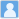• ### Possible evidences from H(z) parameter data for physics beyond Lambda CDM

分类： 天文学 >> 星系和宇宙学 提交时间： 2023-05-24Yang, Rong-Jia

摘要：We analyse H(z) parameter data with some conditions by using Lagrange mean value theorem in Calculus. We find that: (1) there exists at least one decelerated phase at 1 $sigma$ confidence level in the redshift range (0.38, 0.59); (2) the equation of motion of dark energy may be less than -1 at 1 σ confidence level at some redshifts in the redshift range (1.3, 1.53); (3) there exists at least one accelerated phase at 1 σ confidence level in the redshift range (1.037, 1.944). These results may provide possible evidences for physics beyond \$ Lambd ACDM.

同行评议状态:待评议